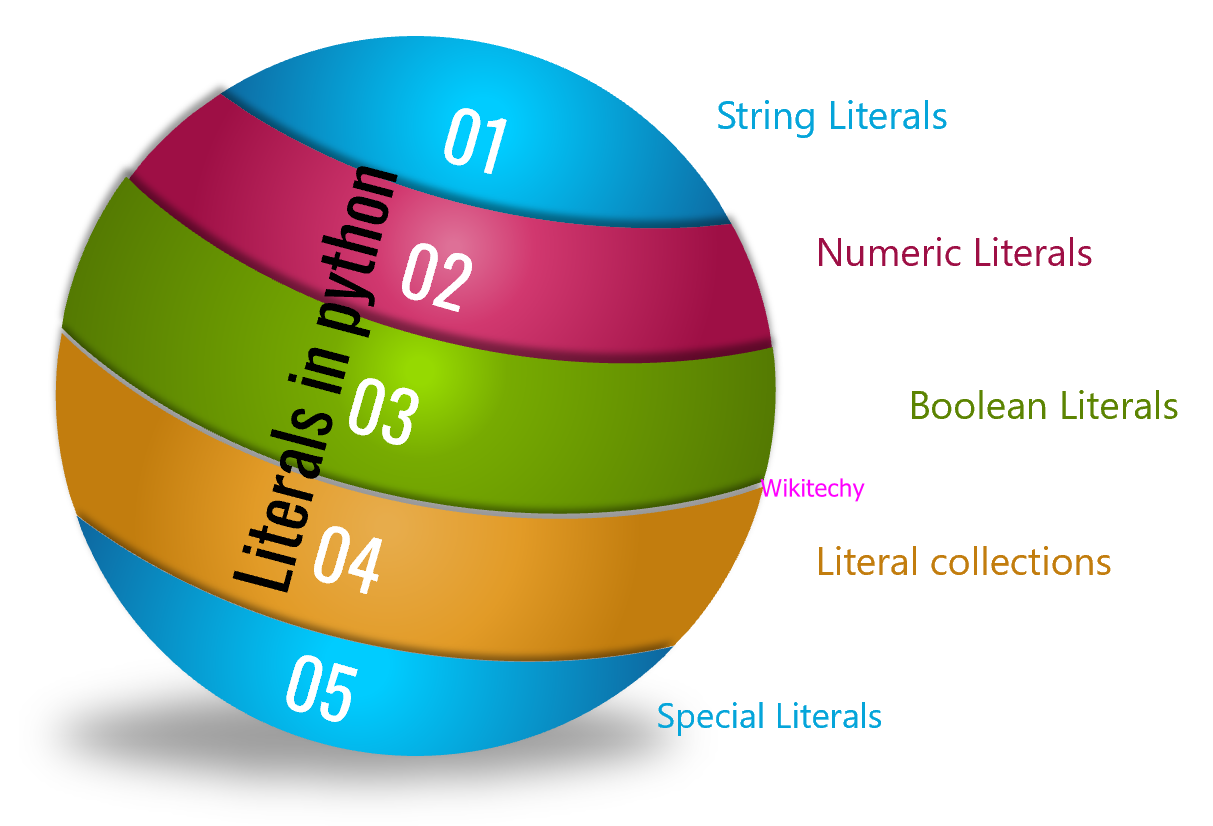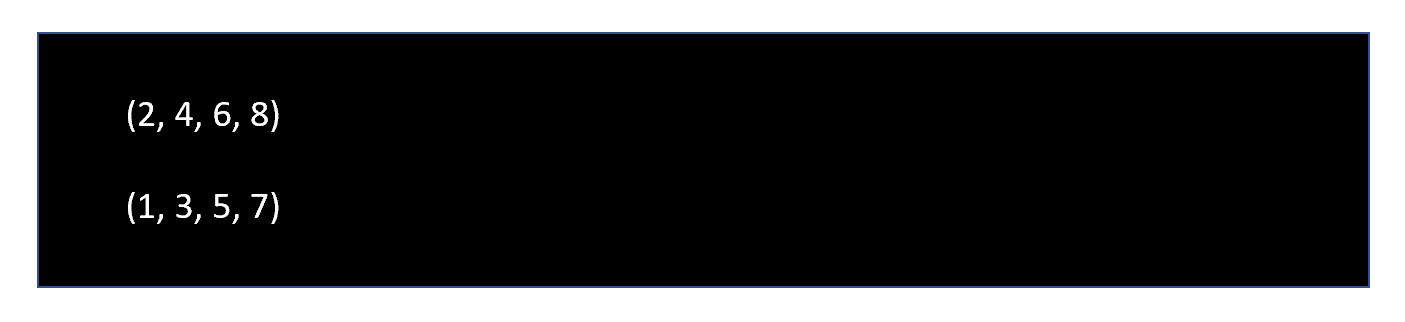# What do you mean by Python literals ?• Literals are a notation for representing a fixed value in source code and it can be defined as raw value or data given in variables or constants.

## String Literals

• A string literal can be created by writing a text with a group of characters surrounded by the single (‘), double (“) or triple quotes.
• We can write multi-line strings or display in the desired way by using triple quotes.

### Sample Code

``````# string literals

# in single quote

s = 'Welcome to Wikitechy'

# in double quotes

t = "Welcome to Wikitechy"

# multi-line String

m = '''Welcome

to

Wikitechy'''

print(s)

print(t)

print(m)``````

### Output## Numeric Literals

• They are immutable and it consists of three data types, they are Integer, Float, Complex.
• In integer both positive and negative numbers including 0 and it should not take any fractional part.
• Float data type are real numbers having both integer and fractional part.

### Sample Code

``````# integer literal

# Binary Literals

a = 0b10100

# Decimal Literal

b = 30

# Octal Literal

c = 0o320

d = 0x12b

print(a, b, c, d)``````

### Output## Boolean Literals

• In python there are only two Boolean literals they are true and false.

### Sample Code

``````x = (1 == True)

y = (2 == False)

z = (3 == True)

r = (1 == True)

a = True + 10

b = False + 10

print("x is", x)

print("y is", y)

print("z is", r)

print("a:", a)

print("b:", b)``````

### Output## Literal Collections

• In this there are four different types of literal collections, they are Lis literals, Tuple literals, Dict literals, Set literals.
• In list literals the values are stored separated by comma (,) and enclosed within square brackets ([ ]).
• Tuple literals are enclosed by the parentheses ‘()‘ and each element is separated by the comma(,) and it is immutable.
• Dictionary literals is enclosed by curly-braces ‘{}‘ and each pair is separated by the commas(,).
• Set literals is the collection of the unordered data set and enclosed by the {} and each element is separated by the comma (,).

### Sample Code

``````# Tuple literals

even_number = (2, 4, 6, 8)

odd_number = (1, 3, 5, 7)

print(even_number)

print(odd_number)``````

### Output## Special Literals

• Python contains one special literal where ‘None’ is used to define a null variable.
• If ‘None’ is compared with anything else other than a ‘None’, it will return false.

### Sample Code

``````# Special literals

water_remain = None

print(water_remain)``````

### Output## What is the Difference between C, C++, Java and Python ?

Answer : Developed Year,1972,1979,1991,1991

## What is Decorator in python ?

Answer : A decorator in Python is a function that takes another function as an argument…

## What is Double Asterisk (**) in python ?

Answer : In Python ** is an exponential operator……

## What is python unsigned right shift (>>>) ?

Answer : The symbol >>> is used to point out that what’s coming after is Python Code…..

## What is Python Wrapper ?

Answer : A wrapper is a python module that interface between python and another software library function which is a non python interface.

## What is Python Networking Programming ?

Answer : The standard library of python supports network protocols….